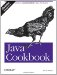# Recipe 6.12 Measuring Elapsed Time

#### Problem

You need to time how long it takes to do something.

#### Solution

Call System.currentTimeMillis( ) twice, or System.nanoTime( ) , and subtract the first result from the second result.

#### Discussion

Needing a timer is such a common thing that, instead of making you depend on some external library, the developers of Java have built it in. The System class contains two static methods for times. currentTimeMillis( ) returns the current time (since 1970) in milliseconds, and nanoTime( ) (new in 1.5) returns the relative time in nanoseconds. To time some event, use this:

`long start = System.currentTimeMillis( );  method_to_be_timed( );  long end = System.currentTimeMillis( ); l ong elapsed = end - start;    // time in milliseconds`

or:

`long start = System.nanoTime( );  method_to_be_timed( );  long end = System.nanoTime( ); l ong elapsed = end - start;    // time in nanoseconds`

Be aware that the millisecond timer works on almost all platforms. The nanosecond timer is always available, but some operating systems and/or hardware combinations may not really provide nanosecond resolution; beware of reading too much into small differences in the values it returns, unless you know that your system is providing, and the JVM is using, a good high-resolution timer.

Here is a short example to measure how long it takes a user to press return. We divide the time in milliseconds by 1,000 to get seconds and print it nicely using a NumberFormat:

`// Timer0.java  long t0, t1;  System.out.println("Press return when ready");  t0=System.currentTimeMillis( );  int b;  do {      b = System.in.read( );  } while (b!='\r' && b != '\n'); t1=System.currentTimeMillis( );  double deltaT = t1-t0;  System.out.println("You took " +       DecimalFormat.getInstance( ).format(deltaT/1000.) + " seconds.");`

This longer example uses the same technique but computes a large number of square roots and writes each one to a discard file. It uses the getDevNull( ) method from Recipe 2.4 to measure how long the computation takes:

`import java.io.*;  import java.text.*;    /**   * Timer for processing sqrt and I/O operations.   */  public class TimeComputation {      public static void main(String argv[]) {          try {              new Timer( ).run( );          } catch (IOException e) {              System.err.println(e);          }      }      public void run( ) throws IOException {            DataOutputStream n = new DataOutputStream(              new BufferedOutputStream(new FileOutputStream(SysDep.getDevNull( ))));          long t0, t1;          System.out.println("Java Starts at " + (t0=System.currentTimeMillis( )));          double k;          for (int i=0; i<100000; i++) {              k = 2.1 * Math.sqrt((double)i);              n.writeDouble(k);          }          System.out.println("Java Ends at " + (t1=System.currentTimeMillis( )));          double deltaT = t1-t0;          System.out.println("This run took " +               DecimalFormat.getInstance( ).format(deltaT/1000.) + " seconds.");      }  }`

This code shows a simpler, but less portable, technique for formatting a " delta t", or time difference. It works only for the English locale (or any other where the number one-and-a-half is written "1.5"), but it's simple enough to write the code inline. I show it here as a method for completeness, and I confess to having used it this way on occasion:

`/** QuickTimeFormat.java - Convert a long ("time_t") to seconds and thousandths. */ public static String msToSecs(long t) {     return Double.toString(t/1000D); }`Java Cookbook, Second Edition
ISBN: 0596007019
EAN: 2147483647
Year: 2003
Pages: 409
Authors: Ian F Darwin

Similar book on Amazon